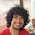### Choose the maximum of two number

Source: P. Winkler

I write two different numbers in the range [a,b], one on each hand. You choose one of my hands at random, I show you the number on that hand. You now guess whether the number you've seen is larger than the number you haven't seen.

Find a strategy for guessing such that, no matter what two numbers I write, you have GREATER THAN a 50% chance of being correct.

Solution:
Highlight the part between the * symbols for the answer.
* I would choose a number randomly in the range [a,b]. Add 0.5 to it and save the number as k in my memory. If the number I see in one hand is less than k, I would say that the number in the other hand is greater. In the other case, I will say that the number in the other hand is less.

One can prove that here, winning probability is greater than 0.5
Let the number that comes out is k'

If k' less than k,
I am doing well when other number is either greater than k or between k' and k

If k' greater than k
I am doing well when other number is either less than k or between k' and k

So, the probability is greater than half, though getting an epsilon is not possible.

Update(05/02/10): Thanx to Aaditya Ramdas for pointing out a "mistake" in the problem (discussion in comments). Problem statement changed slightly and is correct now. :)

1.if I may, this solution is nonsense?!?! (as i found on discussing it :P) As good as saying if the number is positive, keep it (assume the random number u chose in ur head is 0). Is it really true that the probability of a number lying between -N and +infi is different from -N and -infi? :P Kya fart hai :P I guess we all need to learn some basic measure theory first :P

2.@ramdas..
As written in the post, source of the problem and solution is the book by P. Winkler (The only godfather of puzzle alive probably :P).. So, to your question, you cannot say that the solution is non-sense. :P

I also had the same reaction but then it said that the question appeared in some math olympiad. Those guys know measure theory well :P :P

3.just a point to add, the probability that a randomly chosen point in the reals lies in a finite interval (a,b) is _zero_. that is if you can define probability over an interval on the reals (which u cant, I think). so it doesnt even make sense to say "randomly choose a point on the real line" - how? uniformly? u cant! :P anyway, ditch :P

4.@ramdas..
Relabeling the post under unsolved category until someone can answer ur point in a more convincing way :)

Any takers??

5.@Ramdas.. had a discussion with a junior yesterday... came to the conclusion that the point raised by you is indeed correct. Sorry for the trouble. Don't know how Winkler did this mistake. :(

Hence, changing the problem statement to all numbers in the range [a,b]
Thanx

6.That's as good as saying, choose the midpoint of the interval. If one number (the chosen one) lies on one side of the midpoint, then probably the other number lies on the other side, but I'm going to be EVEN safer by saying it is just greater than the picked one. Since it is a uniform distribution of course. Trivial?

There are 4 cases of where the numbers lie wrt the midpoint - LL,RL,LR,RR - each with equal probability. I'm obviously correct in the LR and RL case. Which is 0.5 prob. I'm also correct in the LL case when I chose the smaller of the two. And I'm also correct in the RR case which I chose the larger of the two.

Now, the problem doesn't seem as impressive or as much fun :P

7.if the first number is k, say that the other number is smaller with probability (k-a)/(b-a). i think this is the best probability of winning that u can get and is definitely greater than 50%. eg. a=0, b=10, k=3.
Say that the other number is smaller with probability 30%. you have a 70 % probability of winning.

8.@Ramdas..
Choosing mid point does not work. Note that I have two numbers chosen by me in the two hands. I can choose numbers such that you always lose. Only the hands are chosen by random. :P and not the numbers. So, when you choose k randomly you are doing good. :)

9.Isn't this the same as choosing my threshold to be 0.75? after all my expectation for the limit is 0.75 if I am choosing uniformly.

10.If the chosen number is p say bigger with the probability (p-a)/b-a. If the other number is q, probability of your winning is 1/2*((p-a) + (b-q))/(b-a) [In case q is smaller than p] and 1/2*((b-p) + (q-a))/(b-a) [In case p is smaller than q]. In both cases probability of your winning is 1/2 + 1/2*|p-q|/(b-a)